# Volume of Prisms and Cylinders Warm UP Add

• Slides: 16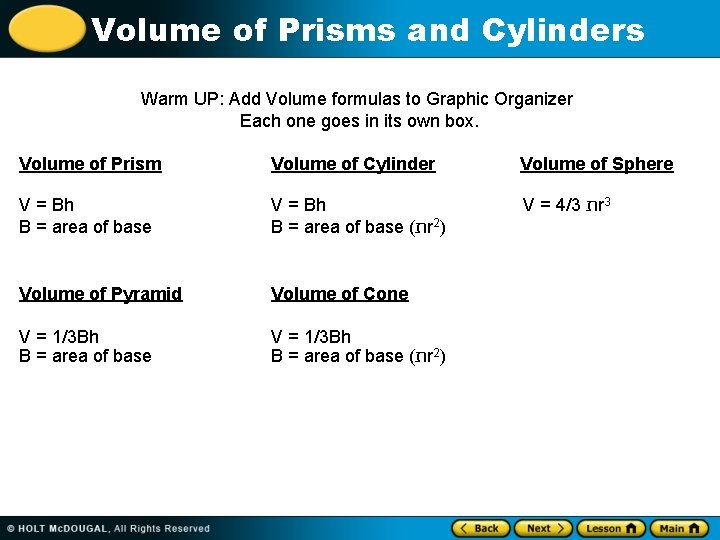Volume of Prisms and Cylinders Warm UP: Add Volume formulas to Graphic Organizer Each one goes in its own box. Volume of Prism Volume of Cylinder Volume of Sphere V = Bh B = area of base ( ת r 2) V = 4/3 ת r 3 Volume of Pyramid Volume of Cone V = 1/3 Bh B = area of base ( ת r 2)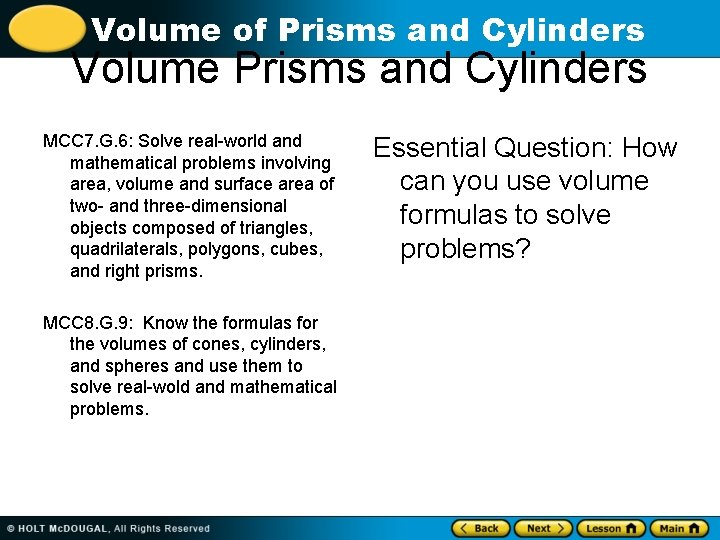Volume of Prisms and Cylinders Volume Prisms and Cylinders MCC 7. G. 6: Solve real-world and mathematical problems involving area, volume and surface area of two- and three-dimensional objects composed of triangles, quadrilaterals, polygons, cubes, and right prisms. MCC 8. G. 9: Know the formulas for the volumes of cones, cylinders, and spheres and use them to solve real-wold and mathematical problems. Essential Question: How can you use volume formulas to solve problems?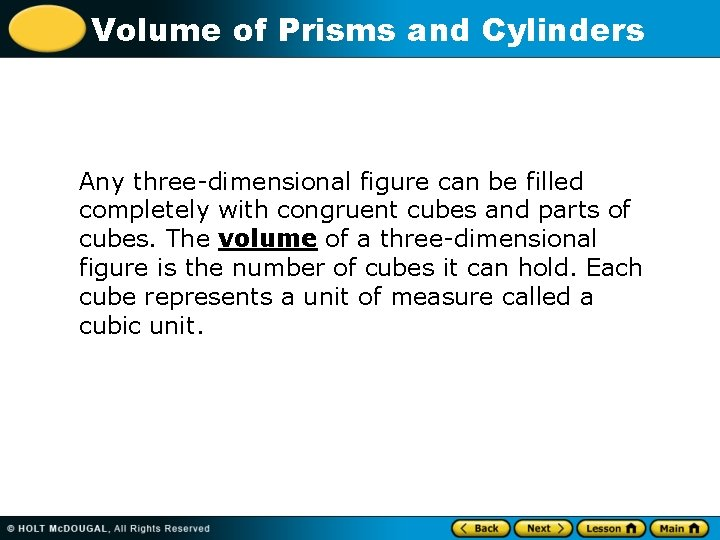Volume of Prisms and Cylinders Any three-dimensional figure can be filled completely with congruent cubes and parts of cubes. The volume of a three-dimensional figure is the number of cubes it can hold. Each cube represents a unit of measure called a cubic unit.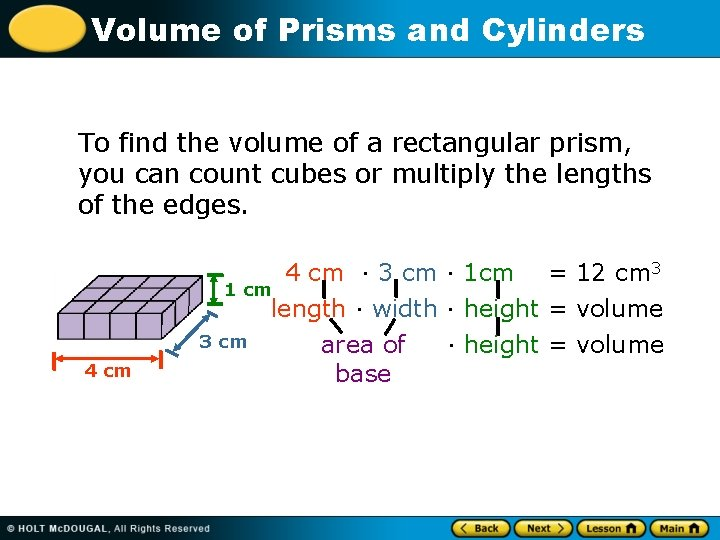Volume of Prisms and Cylinders To find the volume of a rectangular prism, you can count cubes or multiply the lengths of the edges. 4 cm · 3 cm · 1 cm = 12 cm 3 1 cm length · width · height = volume 3 cm area of · height = volume base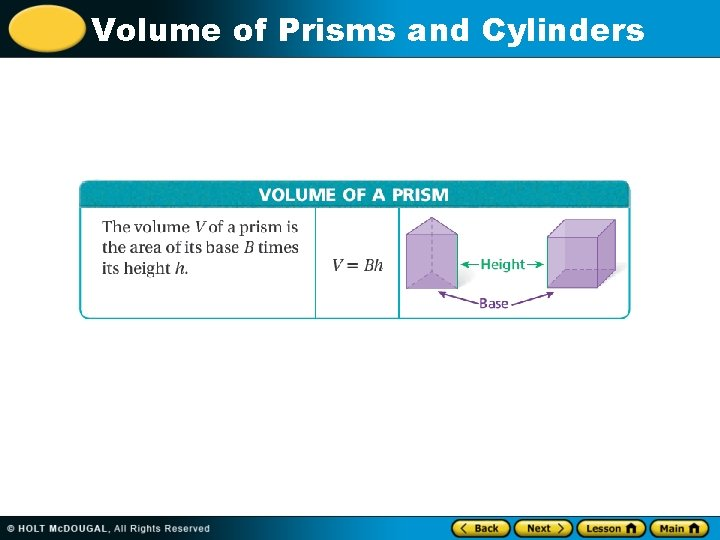Volume of Prisms and Cylinders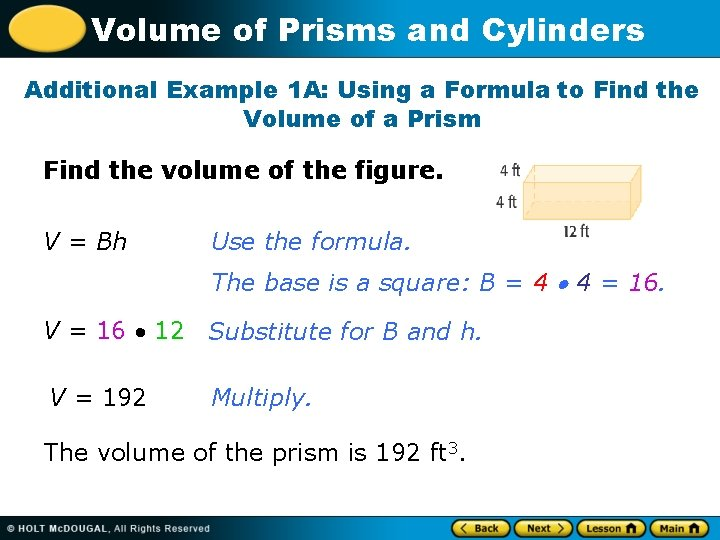Volume of Prisms and Cylinders Additional Example 1 A: Using a Formula to Find the Volume of a Prism Find the volume of the figure. V = Bh Use the formula. The base is a square: B = 4 4 = 16. V = 16 12 Substitute for B and h. V = 192 Multiply. The volume of the prism is 192 ft 3.Volume of Prisms and Cylinders Additional Example 1 B: Using a Formula to Find the Volume of a Prism Find the volume of the figure. V = Bh Use the formula. The base is a triangle: B = 1/2 3 4 = 6. V=6 6 Substitute for B and h. V = 36 Multiply. The volume of the triangular prism is 36 cm 3.Volume of Prisms and Cylinders Check It Out: Example 1 A Find the volume of the figure. V = Bh Use the formula. The base is a square: B = 3 3 = 9. V=9 8 Substitute for B and h. V = 72 Multiply. The volume of the prism is 72 in 3.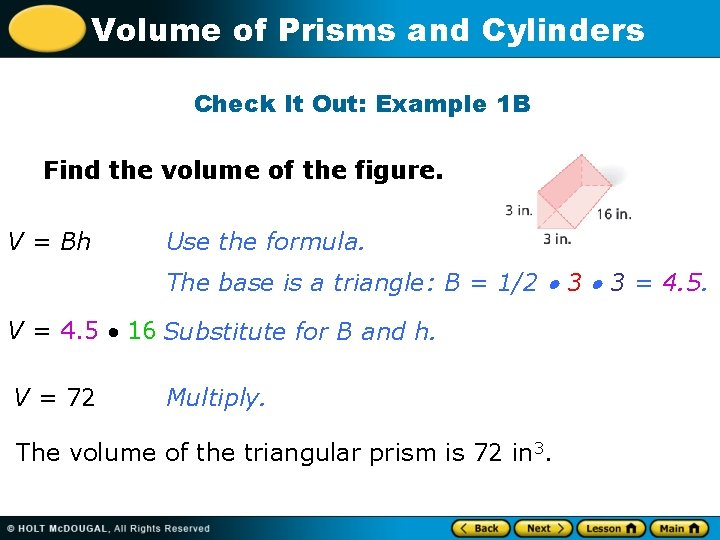Volume of Prisms and Cylinders Check It Out: Example 1 B Find the volume of the figure. V = Bh Use the formula. The base is a triangle: B = 1/2 3 3 = 4. 5. V = 4. 5 16 Substitute for B and h. V = 72 Multiply. The volume of the triangular prism is 72 in 3.Volume of Prisms and Cylinders Reading Math Any unit of measurement with an exponent of 3 is a cubic unit. For example, cm 3 means “cubic centimeter” and in 3 means “cubic inch. ”Volume of Prisms and Cylinders Finding the volume of a cylinder is similar to finding the volume of a prism.Volume of Prisms and Cylinders Additional Example 2: Using a Formula to Find the Volume of a Cylinder A can of tuna is shaped like a cylinder. Find its volume to the nearest tenth. Use 3. 14 for . V = r 2 h Use the formula. The radius of the cylinder is 5 m, and the height is 4. 2 m V 3. 14 · 52 · 4. 2 Substitute for r and h. V 329. 7 Multiply. The volume is about 329. 7 m 3.Volume of Prisms and Cylinders Check It Out: Example 2 Find the volume of a cylinder to the nearest tenth. Use 3. 14 for . 7 m 3. 8 m V = r 2 h Use the formula. The radius of the cylinder is 7 m, and the height is 3. 8 m V 3. 14 · 72 · 3. 8 Substitute for r and h. V 584. 668 Multiply. The volume is about 584. 7 m 3.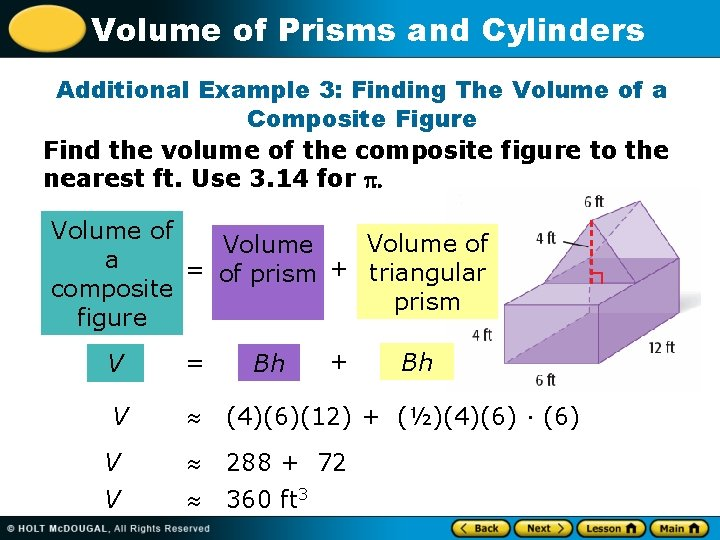Volume of Prisms and Cylinders Additional Example 3: Finding The Volume of a Composite Figure Find the volume of the composite figure to the nearest ft. Use 3. 14 for . Volume of Volume a = of prism + triangular composite prism figure + V = V (4)(6)(12) + (½)(4)(6) · (6) V 288 + 72 V 360 ft 3 Bh BhVolume of Prisms and Cylinders Check It Out: Example 3 Find the volume of the composite figure to the nearest ft. Use 3. 14 for . Volume of Volume a = of prism + composite figure + Volume of triangular prism 10 ft V = V (4)(5)(10) + (½)(4)(5) · (6) V 200 + 60 V 260 ft 3 Bh Bh 5 ft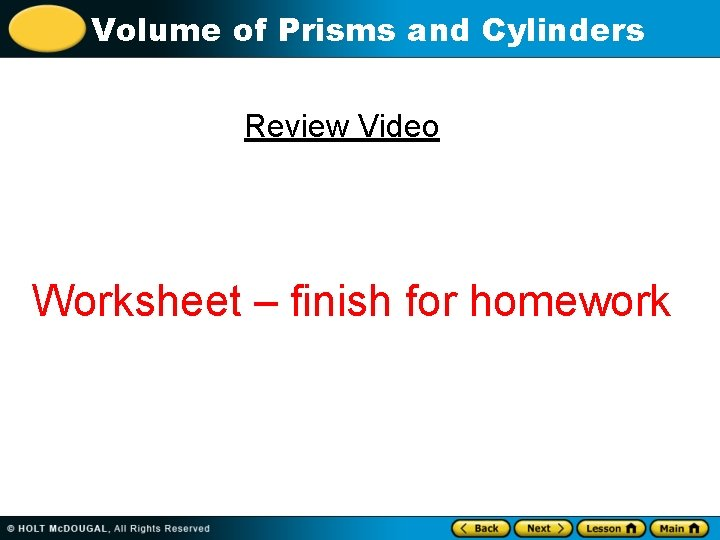Volume of Prisms and Cylinders Review Video Worksheet – finish for homework